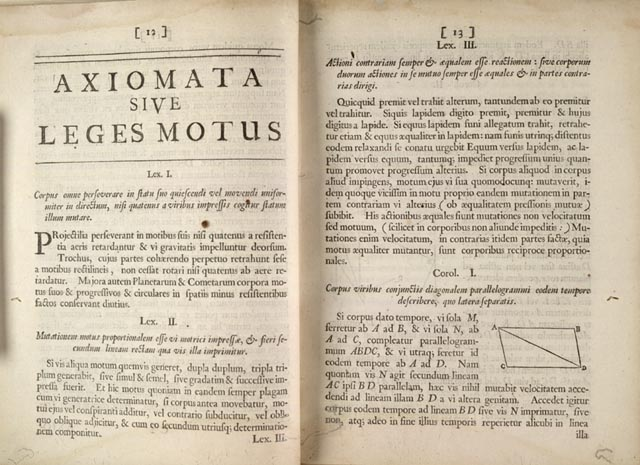Newton's laws and other laws of nature cannot be "derived" or "proved" like proofs in Math. Instead, physical laws are conjectured and then studied through experiments. These laws can be verified under different limits, in which case the conjected laws become accepted theory, or they can be falsified in the experiments, in which case the conjectured can be trashed.

For example, during the time of Aristotle, it was commonly thought that the free fall speed of an object is proportional to its weight, namely, heavier objects fall faster. Another misconception was the Aristotlian idea that in the absence of any external motive power, all objects (on Earth) would naturally come to rest, to a state of no motion. These ideas and others would have been easily falsified if the Greeks and their later followers would have realized that natural laws should be experimentally verified. For us, this concept is almost trivial, but it was only Francis Bacon in the 16th century who solidified this idea.

Newton derived his laws following his analysis of different experiments and observations, but later his laws were verified time and again in many different experimental setups. It is only once we leave the realm of classical mechanics that we have to modify Newton's laws. Special Relativity is required for very high speeds while very small scales requires quantum mechanics.

### Newton's First law of Motion: Law of Inertia

The first law, which is called the law of intertia, was to some extent first formulated by Galileo, who realized that:
“ A body moving on a level surface will continue in the same direction at a constant speed unless disturbed.”

Newton later reformulated as a somewhat more general conjecture:
First Law of Motion: Unless acted upon by an unbalanced force, an object will maintain a constant velocity.

In other words, an object at rest will stay at rest, while an object in motion will tend to stay in motion in a straight line while keeping a constant velocity, unless acted upon by an external force.

### Newton's Second law of Motion: Law of Motion

Loosly translated from latin, Newton's second law of motion states that:
Second Law of Motion: The change of motion is always proportional to the motive force impressed; and is made in the direction of the right line in which that force is impressed.

That is, Newton's original formulation of his second law in current day notation is
$${d{\bf v} \over dt} \propto {\bf F}$$
Newton knew also very well that the change of motion is large for smaller masses, namely, that
$$F \propto m {d{\bf v} \over dt}$$
Newton did not use the concept of momentum, which was developed only later by Leibniz (the "quantité d'avancement"). For Newton, mass was constant, so the derivative of v or the derivative of p=mv, could not make a big difference anyway. However, once mass is allowed to vary (such as a rocket, or in the theory of relativity), the correct form of the law is in fact that the force is proportional to the rate of change of the momentum:
$$F \propto {d{\bf p} \over dt} = {d(m{\bf v}) \over dt}.$$
What about the factor in the proportionality? Well, it turns out that we are free to choose whichever factor we wish! This is because we haven't stated with which units we measure the force! In fact, we choose the proportionality constant to be unity and with that define the units of force. Thus, in SI, the unit of force called Newton is defined as kg m s-2. In c.g.s, the unit is: dyne = gr cm s-2.

### Newton's Third law of Motion: Law of Action and Reaction

Newton's third law states that
Third Law of Motion: All forces occur in pairs, and these two forces are equal in magnitude and opposite in direction.

To every force applied there is an equal but opposite force applied (i.e., in the opposite direction). In other words, if object A exerts a force on object B, object B will exert the same magnitude force on A, but in the opposite direction.Newton's three laws of motion. A translation dating back to 1729 can be found here.

## Blogroll

Sensible Climate
Physics and more Other